# Compute private statistics with PipelineDP

## 1. Before you begin

You might think that aggregate statistics don't leak any information about the individuals to whom they pertain. However, there are many ways that an attacker can learn sensitive information about individuals from aggregate statistics.

In this codelab, you learn how to produce private statistics with differentially private aggregations from PipelineDP to protect individuals' privacy. PipelineDP is a Python framework that lets you apply differential privacy to large datasets with batch-processing systems, such as Apache Spark and Apache Beam. For more information about how to compute differentially private statistics in Go, see the Privacy on Beam codelab.

Private means that the output is produced in a way that doesn't leak any private information about the individuals in the data. You can achieve this outcome through differential privacy, a strong privacy notion of anonymization, which is the process of data aggregation across multiple users to protect user privacy. All anonymization methods use aggregation, but not all aggregation methods achieve anonymization. Differential privacy, on the other hand, provides measurable guarantees about information leakage and privacy.

## Prerequisites

• Familiarity with Python
• Familiarity with basic data aggregation
• Experience with pandas, Spark, and Beam

## What you'll learn

• The basics of differential privacy
• How to calculate differentially private summary statistics with PipelineDP
• How to tweak your results with additional privacy and utility parameters

## What you'll need

• If you want to run the codelab in your own environment, you need Python 3.7 or higher installed on your computer.
• If you want to follow the codelab without your own environment, you need access to Colaboratory.

## 2. Understand differential privacy

To better understand differential privacy, look at this simple example.

Imagine that you work in the marketing department of an online fashion retailer and you want to understand which of your products are most likely to sell.

This chart shows which products the customers looked at first when they visited the shop's website: t-shirts, jumpers, socks, or jeans. T-shirts are the most popular item while socks are the least popular item.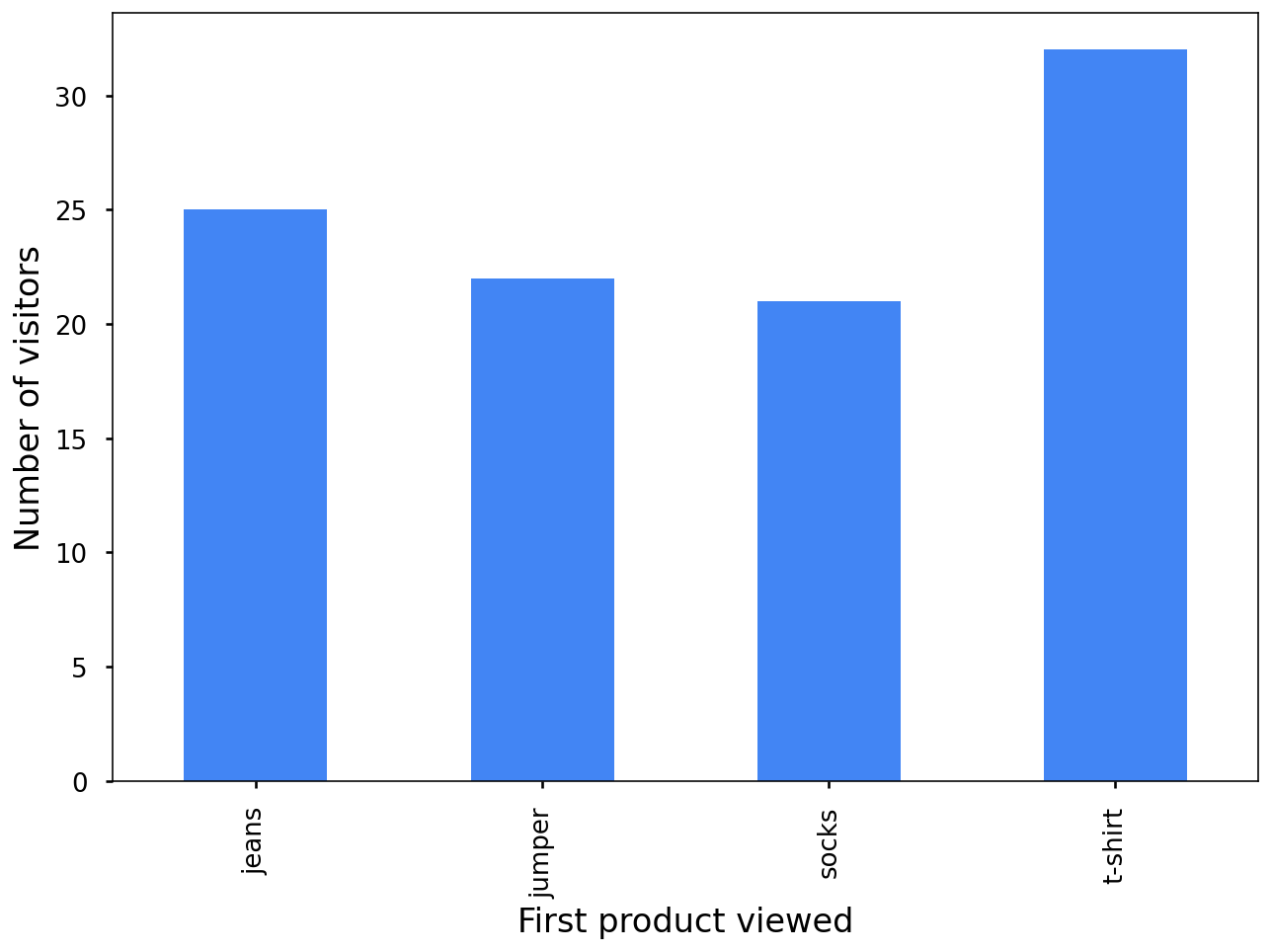This looks useful, but there's a catch. When you want to take additional information into account, such as whether customers made a purchase or which product they viewed second, you risk revealing individuals in your data.

This chart shows you that only one customer looked at a jumper first and then actually made a purchase: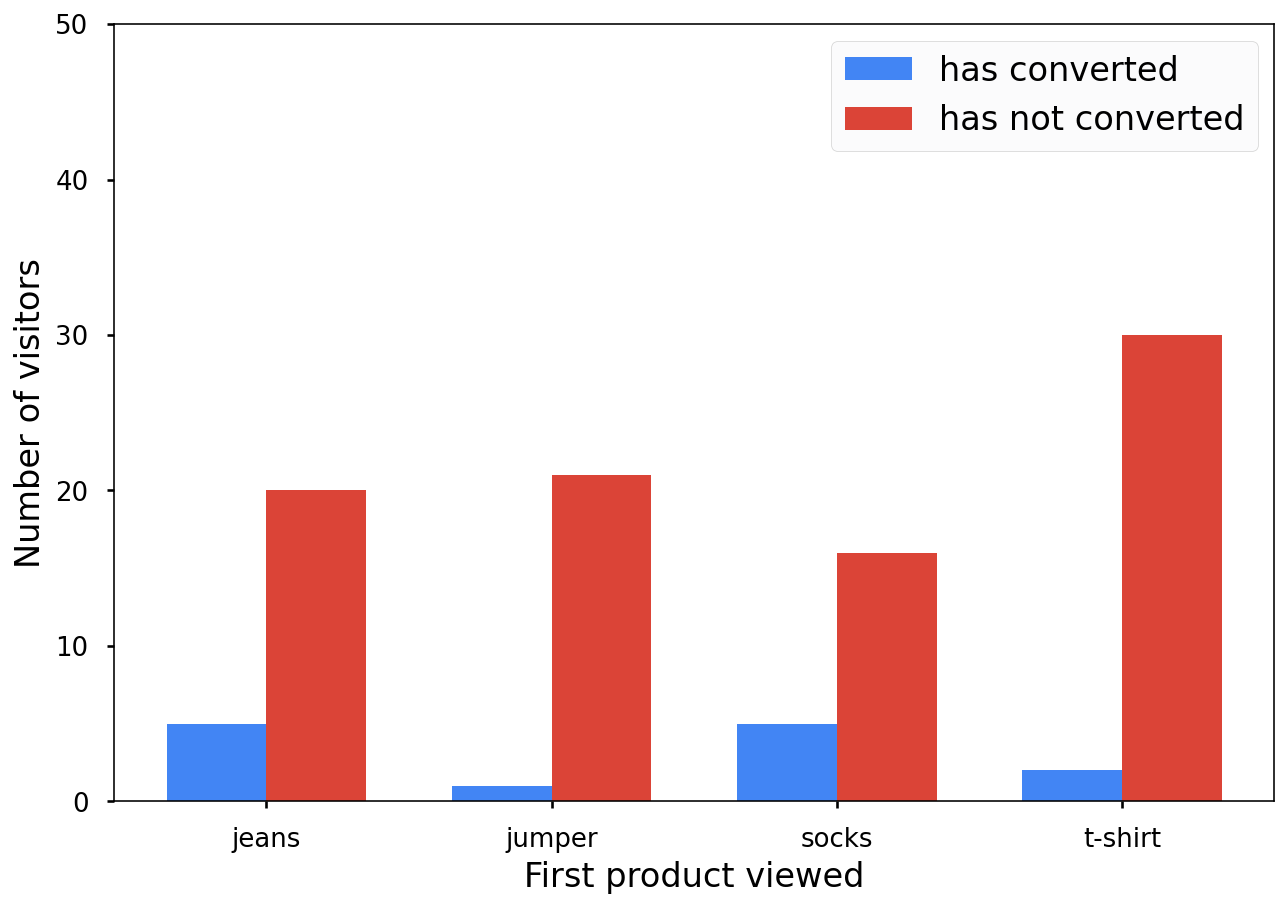This isn't great from a privacy perspective. Anonymized statistics shouldn't reveal individual contributions, so what do you do? You add random noise to your bar charts to make them a bit less accurate!

This bar chart isn't entirely accurate, but it's still useful and it doesn't reveal individual contributions: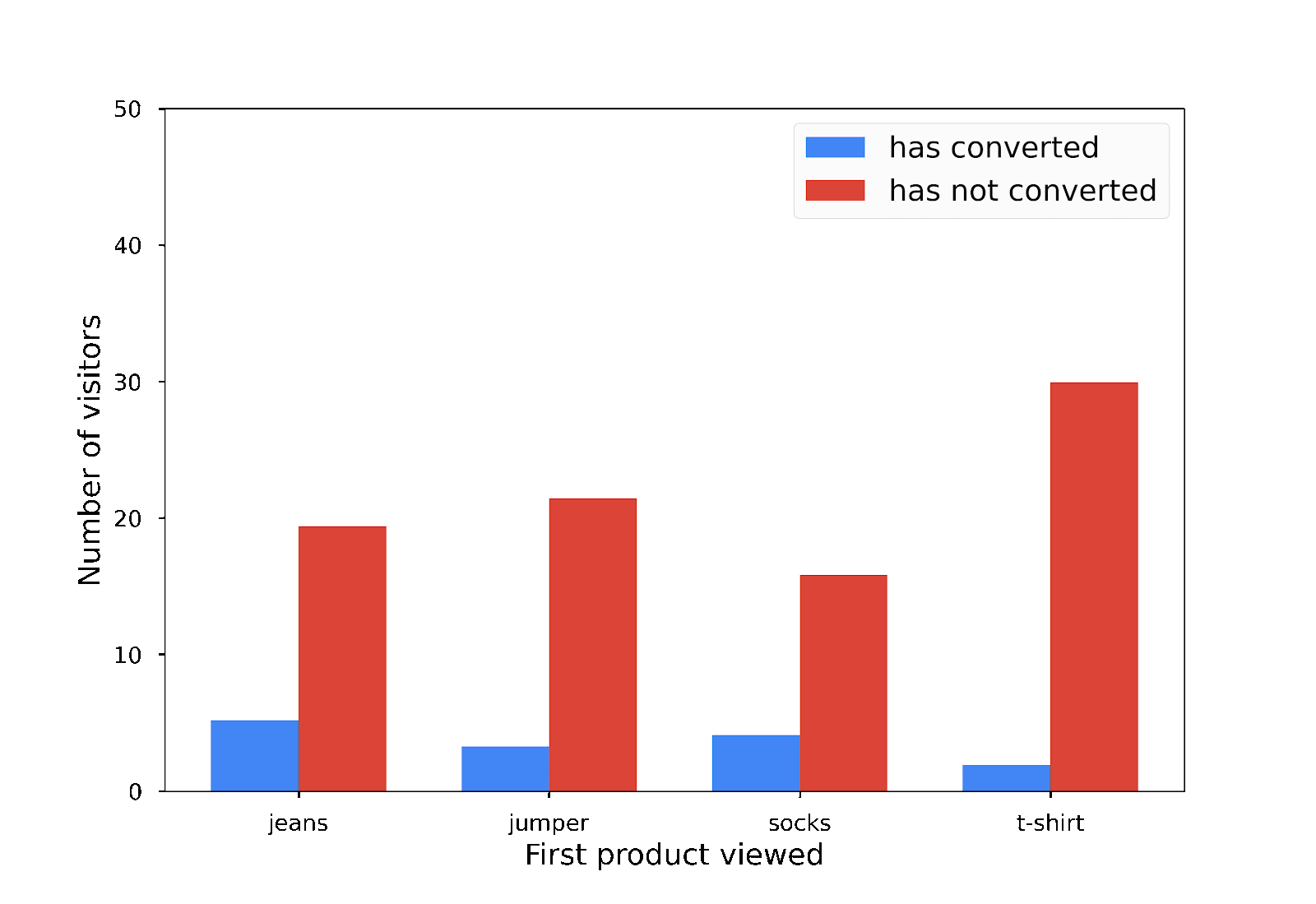Differential privacy is the addition of the right amount of random noise to mask individual contributions.

This example is oversimplified. The proper implementation of differential privacy is more involved and comes with a number of unexpected implementation subtleties. Similar to cryptography, it might not be a great idea to create your own implementation of differential privacy. Instead, you can use PipelineDP.

You don't need to install PipelineDP follow this codelab because you can find all the relevant code and graphs in this document.

To play with PipelineDP, run it yourself, or use it later:

```pip install pipeline-dp
```

If you want to run the example using Apache Beam:

```pip install apache_beam
```

You can find the code for this codelab and the dataset in the `PipelineDP/examples/codelab/` directory.

## 4. Compute conversion metrics per first product viewed

Imagine that you work at an online fashion retailer and you want to understand which of your different product categories generate the highest number and value of conversions when viewed first. You want to share this information with your marketing agency as well as other internal teams, but you want to prevent the leak of information about any individual customer.

To compute conversion metrics per first product viewed for the website:

1. Review the mock dataset of visits to your website in the `PipelineDP/examples/codelab/` directory.

This screenshot is an example of the dataset. It contains the user's ID, the products that a user viewed, whether the visitor converted, and, if so, the value of the conversion.

 user_id product_view_0 product_view_1 product_view_2 product_view_3 product_view_4 has_conversion conversion_value 0 jeans t_shirt t_shirt none none false 0.0 1 jeans t_shirt jeans jumper none false 0.0 2 t_shirt jumper t_shirt t_shirt none true 105.19 3 t_shirt t_shirt jeans none none false 0.0 4 t_shirt socks jeans jeans none false 0.0

You're interested in these metrics:

• `view_counts`: The number of times that your website's visitors see each product first.
• `total_conversion_value`: The total amount of money that visitors spend when they convert.
• `conversion_rate`: The rate at which visitors convert.
1. Generate the metrics in a non-private way:
``````conversion_metrics = df.groupby(['product_view_0'
])[['conversion_value', 'has_conversion']].agg({
'conversion_value': [len, np.sum],
'has_conversion': np.mean
})
conversion_metrics = conversion_metrics.rename(
columns={
'len': 'view_counts',
'sum': 'total_conversion_value',
'mean': 'conversion_rate'
}).droplevel(
0, axis=1)
``````

As you learned earlier, these statistics can reveal information about individuals in your dataset. For instance, only one person converted after the person saw a jumper first. For 22 views, your conversion rate is approximately 0.05. Now you need to transform each bar chart into a private one.

1. Define your privacy parameters with the `pipeline_dp.NaiveBudgetAccountant` class, and then specify the `epsilon` and `delta` arguments that you want to use for your analysis.

How you set these arguments depends on your particular problem. To learn more about them, see Optional: Tweak the differential-privacy parameters.

This code snippet uses example values:

``````budget_accountant = pipeline_dp.NaiveBudgetAccountant(
total_epsilon=1, total_delta=1e-5)
``````
1. Initialize the `LocalBackend` instance:
``````ops = pipeline_dp.LocalBackend()
``````

You can use the `LocalBackend` instance because you run this program locally without additional frameworks, such as Beam or Spark.

1. Initialize the `DPEngine` instance:
``````dp_engine = pipeline_dp.DPEngine(budget_accountant, ops)
``````

PipelineDP lets you specify further parameters through the `pipeline_dp.AggregateParams` class, which affects the generation of your private statistics.

``````params = pipeline_dp.AggregateParams(
noise_kind=pipeline_dp.NoiseKind.LAPLACE,
metrics=[pipeline_dp.Metrics.COUNT],
max_partitions_contributed=1,
max_contributions_per_partition=1)
``````
1. Specify that you want to calculate the `count` metric and use the `LAPLACE` noise distribution.
2. Set the `max_partitions_contributed` argument to a `1` value.

This argument bounds how many different visits a user can contribute. You expect users to visit the website once per day and you don't care whether they visit it multiple times over the course of the day.

1. Set the `max_contributions_per_partitions` argument to a `1` value.

This argument specifies how many contributions a single visitor can make to an individual partition or a product category in this case.

1. Create a `data_extractor` instance that specifies where to find the `privacy_id`, `partition`, and `value` fields.

Your code should look like this code snippet:

``````def run_pipeline(data, ops):
budget_accountant = pipeline_dp.NaiveBudgetAccountant(
total_epsilon=1, total_delta=1e-5)

dp_engine = pipeline_dp.DPEngine(budget_accountant, ops)

params = pipeline_dp.AggregateParams(
noise_kind=pipeline_dp.NoiseKind.LAPLACE,
metrics=[pipeline_dp.Metrics.COUNT],
max_partitions_contributed=1, # A single user can only contribute to one partition.
max_contributions_per_partition=1, # For a single partition, only one contribution per user is used.
)

data_extractors = pipeline_dp.DataExtractors(
privacy_id_extractor=lambda row: row.user_id,
partition_extractor=lambda row: row.product_view_0
value_extractor=lambda row: row.has_conversion)

dp_result = dp_engine.aggregate(data, params, data_extractors)

budget_accountant.compute_budgets()

return dp_result
``````
1. Add this code to transform your Pandas DataFrame into a list of rows from which you can directly calculate differentially private statistics:
``````rows = [index_row for index_row in df.iterrows()]
dp_result_local = run_pipeline(rows, ops) # Returns generator
list(dp_result_local)
``````

Congratulations! You calculated your first differentially private statistic!

This chart shows the result of your differentially private count next to the non-private count that you calculated earlier: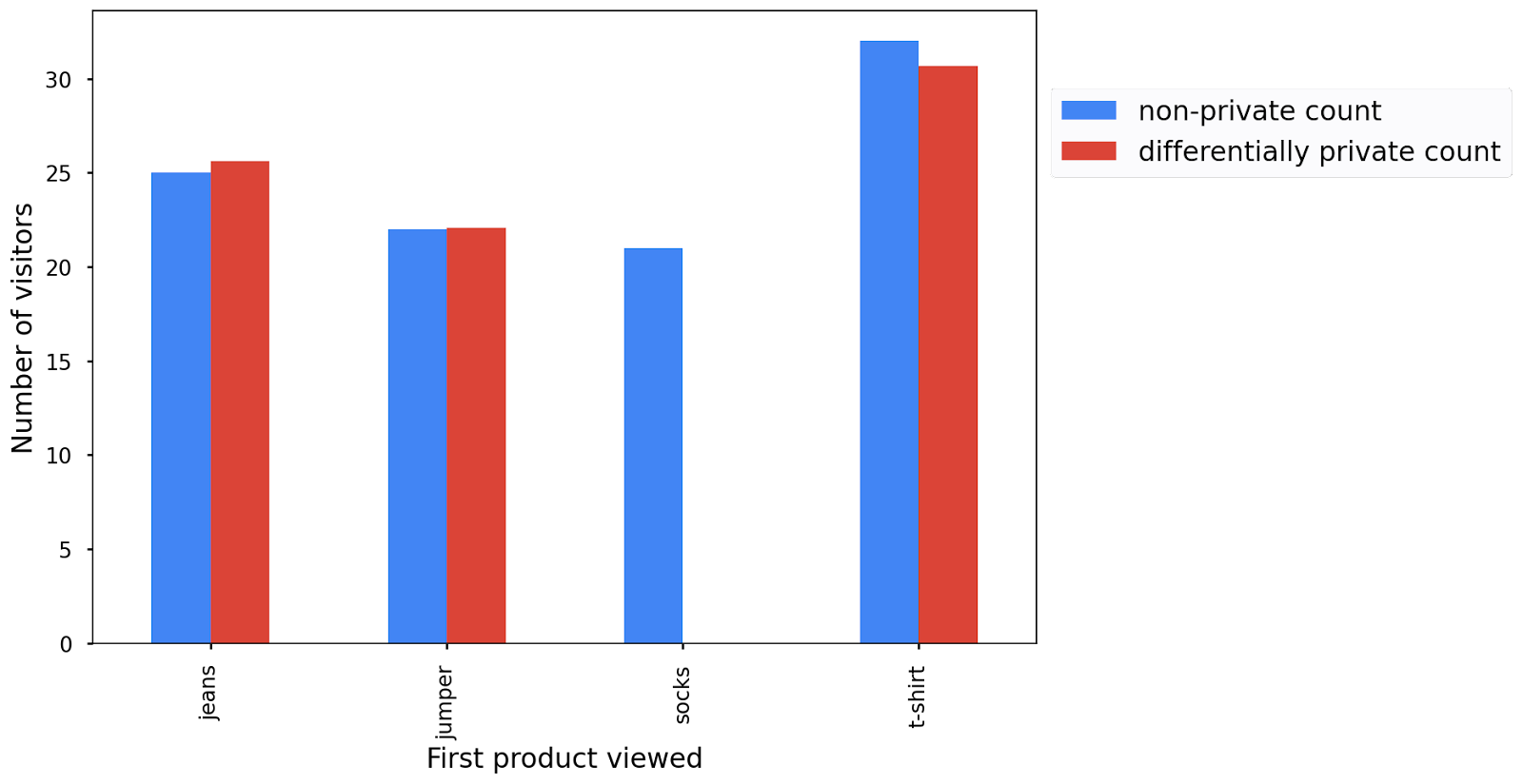The bar chart that you get when you run the code might differ from this one, which is okay. Due to the noise in differential privacy, you get a different bar chart each time that you run the code, but you can see that they're similar to the original non-private bar chart.

Please note that it's very important for the privacy guarantees to not run the pipeline multiple times for the sake of privacy guarantees. For more information, see Compute multiple statistics.

## 5. Use public partitions

In the previous section, you might have noticed that you dropped all visits data for a partition, namely visitors that first saw socks on your website.

This is due to partition selection or thresholding, an important step to ensure differential-privacy guarantees when the existence of output partitions depends on the user data itself. When this is the case, the mere existence of a partition in the output can leak the existence of an individual user in the data. To learn more about why this violates privacy, see this blog post. To prevent this privacy violation, PipelineDP only keeps partitions with a sufficient number of users in them.

When the list of output partitions doesn't depend on private user data, you don't need this partition-selection step. This is actually the case for your example because you know all possible product categories that a customer could see first.

To use partitions:

1. Create a list of your possible partitions:
``````public_partitions_products = ['jeans', 'jumper', 'socks', 't-shirt']
``````
1. Pass the list to the `run_pipeline()` function, which sets it as an additional input to the `pipeline_dp.AggregateParams` class:
``````run_pipeline(
rows, ops, total_delta=0, public_partitions=public_partitions_products)
# Returns generator
``````
``````params = pipeline_dp.AggregateParams(
noise_kind=pipeline_dp.NoiseKind.LAPLACE,
metrics=[pipeline_dp.Metrics.COUNT],
max_partitions_contributed=1,
max_contributions_per_partition=1,
public_partitions=public_partitions_products)
``````

If you use public partitions and `LAPLACE` noise, it's possible to set the `total_delta` argument to a `0` value.

Now you see in the result that data for all partitions, or products, is reported.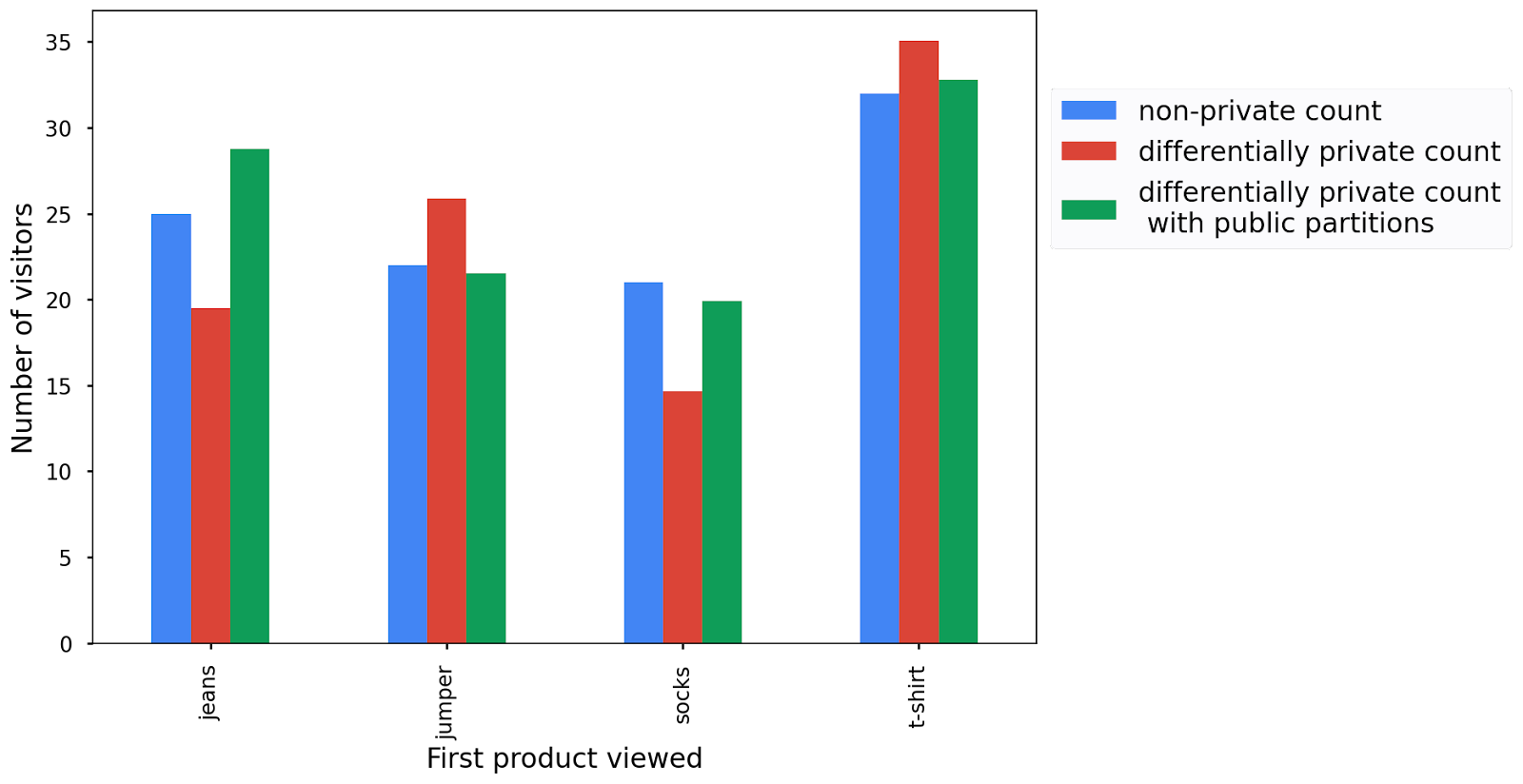Not only do public partitions let you keep more partitions, but they also add roughly half as much noise because you don't spend any privacy budget on partition selection, so the difference between raw and private counts is slightly less compared to the previous run.

There are two important things to keep in mind when you use public partitions:

• Be careful when you derive the list of partitions from raw data. If you don't do this in a differentially private way, your pipeline no longer provides differential privacy guarantees. For more information, see Advanced: Derive partitions from data.
• If there's no data for some of the public partitions, you need to apply noise to those partitions to preserve differential privacy. For example, if you used an additional product like trousers, which doesn't occur in your dataset or on your website, it's still noise and the results might show some visits to products when there were none.

## Advanced: Derive partitions from data

If you run multiple aggregations with the same list of non-public output partitions in the same pipeline, you can derive the list of partitions once with the `dp_engine.select_private_partitions()` method and supply the partitions to each aggregation as the `public_partitions` input. Not only is this safe from a privacy perspective, but it also lets you add less noise because you only use the privacy budget on partition selection once for the entire pipeline.

``````def get_private_product_views(data, ops):
"""Obtains the list of product_views in a private manner.

This does not calculate any private metrics; it merely obtains the list of
product_views but does so while making sure the result is differentially private.
"""

# Set the total privacy budget.
budget_accountant = pipeline_dp.NaiveBudgetAccountant(
total_epsilon=1, total_delta=1e-5)

# Create a DPEngine instance.
dp_engine = pipeline_dp.DPEngine(budget_accountant, ops)

# Specify how to extract privacy_id, partition_key, and value from a
# single element.
data_extractors = pipeline_dp.DataExtractors(
partition_extractor=lambda row: row.product_view_0,
privacy_id_extractor=lambda row: row.user_id)

# Run aggregation.
dp_result = dp_engine.select_partitions(
data, pipeline_dp.SelectPrivatePartitionsParams(
max_partitions_contributed=1),
data_extractors=data_extractors)

budget_accountant.compute_budgets()
return dp_result
``````

## 6. Compute multiple statistics

Now that you know how PipelineDP works, you can see how you can use it for some more advanced use cases. As mentioned at the beginning, you're interested in three statistics. PipelineDP lets you compute multiple statistics at the same time as long as those share the same parameters in the `AggregateParams` instance, which you see later. Not only is it cleaner and easier to calculate multiple metrics in one go, it's also better in terms of privacy.

If you remember the `epsilon` and `delta` parameters that you supply to the `NaiveBudgetAccountant` class, they represent something called a privacy budget, which is a measure of the amount of user privacy that you leak from the data.

An important thing to remember about the privacy budget is that it's additive. If you run a pipeline with a particular epsilon ε and delta δ a single time, you spend an (ε,δ) budget. If you run it a second time, you spend a total budget of (2ε, 2δ). Similarly, if you compute multiple statistics with a `NaiveBudgetAccountant` method and consecutively a privacy budget of ε,δ, you spend a total budget of (2ε, 2δ). This means that you degrade the privacy guarantees.

To circumvent this, you need to use a single `NaiveBudgetAccountant` instance with the total budget that you want to use when you need to compute multiple statistics over the same data. You then need to specify the `epsilon` and `delta` values that you want to use for each aggregation. In the end, you end up with the same overall privacy guarantee, but the higher `epsilon` and `delta` values that a particular aggregation has, the higher accuracy it has.

To see this in action, you can compute the `count`, `mean`, and `sum` statistics.

You calculate statistics on top of two different metrics: a `conversion_value` metric, which you use to infer the amount of revenue generated based on which product is viewed first, and a `has_conversion` metric, which you use to calculate the number of visitors to your website and the average conversion rate.

For each metric, you need to separately specify the parameters that guide the calculation of the private statistics. You split your privacy budget across the two metrics. You calculate two statistics from the `has_conversion` metric, so you want to assign it two-thirds of your initial budget and assign the other one-third to the `conversion_value` metric.

To compute multiple statistics:

1. Set up your privacy budget accountant with the total `epsilon` and `delta` values that you want to use across the three statistics:
``````budget_accountant = pipeline_dp.NaiveBudgetAccountant(
total_epsilon=1, total_delta=0)
``````
1. Initialize the `DPEngine` to calculate your metrics:
`````` dp_engine = pipeline_dp.DPEngine(budget_accountant, ops)
``````
1. Specify the parameters for this metric.
``````params_conversion_value_metrics = pipeline_dp.AggregateParams(
noise_kind=pipeline_dp.NoiseKind.LAPLACE,
metrics=[pipeline_dp.Metrics.SUM],
max_partitions_contributed=1,
max_contributions_per_partition=1,
min_value=0,
max_value=100,
public_partitions=public_partitions,
budget_weight=1/3)
``````

The last argument optionally specifies the weight of your privacy budget. You could give the same weight to all, but you want to set this argument to one-third as explained earlier.

You also set a `min_value` and `max_value` argument to specify the lower and upper bound applied to a value contributed by a unit of privacy in a partition. These parameters are required when you want to calculate a private sum or mean. You don't expect negative values, so you can assume `0` and `100` as reasonable bounds.

1. Extract the relevant data and then pass it to the aggregation function:
``````data_extractors_conversion_value_metrics = pipeline_dp.DataExtractors(
privacy_id_extractor=lambda row: row.user_id,
partition_extractor=lambda row: row.product_view_0,
value_extractor=lambda row: row.conversion_value)

dp_result_conversion_value_metrics = (
dp_engine.aggregate(data, params_conversion_value_metrics,
data_extractors_conversion_value_metrics))
``````
1. Follow the same steps to calculate the two metrics based on your `has_conversion` variable:
``````params_conversion_rate_metrics = pipeline_dp.AggregateParams(
noise_kind=pipeline_dp.NoiseKind.LAPLACE,
metrics=[pipeline_dp.Metrics.COUNT, pipeline_dp.Metrics.MEAN],
max_partitions_contributed=1,
max_contributions_per_partition=1,
min_value=0,
max_value=1,
public_partitions=public_partitions,
budget_weight=2/3)

data_extractors_conversion_rate_metrics = pipeline_dp.DataExtractors(
privacy_id_extractor=lambda row: row.user_id,
partition_extractor=lambda row: row.product_view_0,
value_extractor=lambda row: row.has_conversion)

dp_result_conversion_rate_metrics = (
dp_engine.aggregate(data, params_conversion_rate_metrics,
data_extractors_conversion_rate_metrics))
``````

The only change is in the `pipeline_dp.AggregateParams` instance, in which you now define `mean` and `count` as aggregations, and assign two-thirds of your privacy budget to this calculation. Because you want to have the same contribution bounds for both statistics and calculate them on top of the same `has_conversion` variable, you can combine them in the same `pipeline_dp.AggregateParams` instance and calculate them at the same time.

1. Call the `budget_accountant.compute_budgets()` method:
``````budget_accountant.compute_budgets()
``````

You can plot all three private statistics in comparison to their original statistics. Depending on the noise added, you see that the results can actually fall outside of the plausible scale. In this instance, you see a negative conversion rate and total conversion value for jumpers because the noise added is symmetric around zero. For further analyses and processing, it's best to not manually post-process the private statistics, but if you wanted to add those plots to a report, you could simply set the minimum to zero afterward without violation of the privacy guarantees.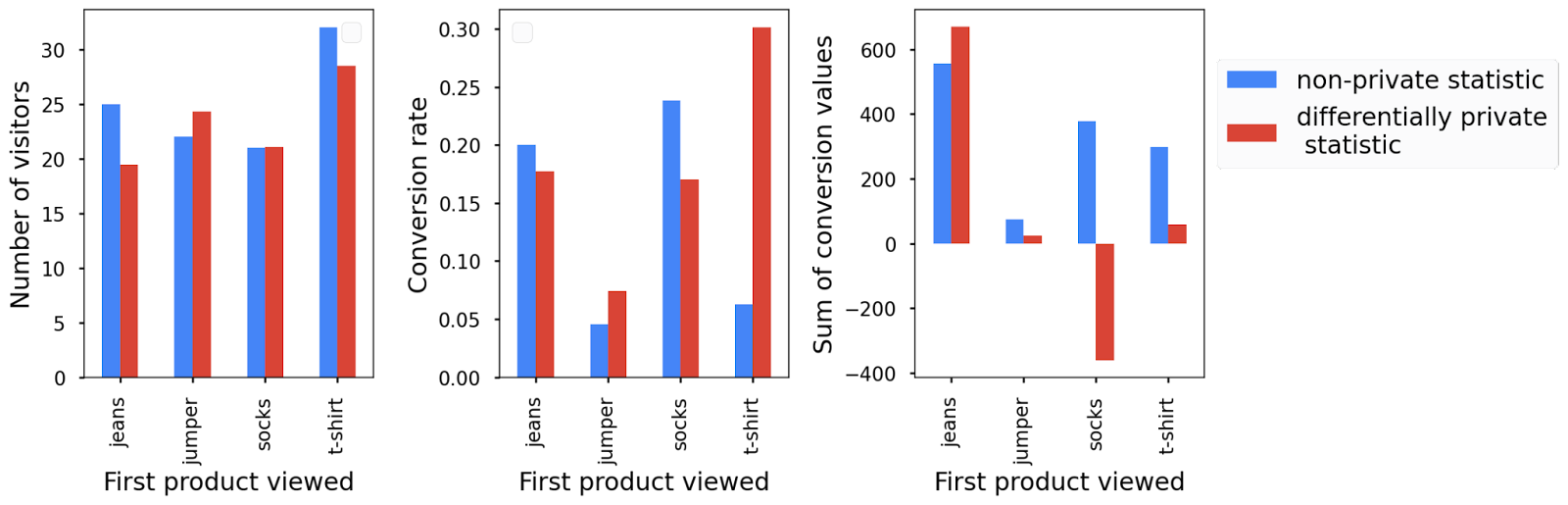## 7. Run the pipeline with Beam

Data processing nowadays requires you to deal with huge amounts of data, so much so that you can't process it locally. Instead, many people use frameworks for large-scale data processing, such as Beam or Spark, and run their pipelines in the cloud.

PipelineDP supports Beam and Spark with only small changes to your code.

To run the pipeline with Beam with the `private_beam` API:

1. Initialize a `runner` variable and then create a pipeline in which you apply your privacy operations to a Beam representation of your `rows`:
``````runner = fn_api_runner.FnApiRunner()  # local runner

with beam.Pipeline(runner=runner) as pipeline:
beam_data = pipeline | beam.Create(rows)
``````
1. Create a `budget_accountant` variable with your required privacy parameters:
``````budget_accountant = pipeline_dp.NaiveBudgetAccountant(
total_epsilon=1, total_delta=0)
``````
1. Create a `pcol`, or private collection, variable, which guarantees that any aggregations conform with your privacy requirements:
``````pcol = beam_data | pbeam.MakePrivate(
budget_accountant=budget_accountant,
privacy_id_extractor=lambda
row: row.user_id)
``````
1. Specify the parameters of your private aggregation in the appropriate class.

Here, you use the `pipeline_dp.aggregate_params.SumParams()`class because you calculate the sum of product views.

1. Pass your aggregation parameters to `pbeam.Sum` method to calculate your statistic:
``````dp_result = pcol | pbeam.Sum(params)
``````
1. In the end, your code should look like this code snippet:
``````import pipeline_dp.private_beam as pbeam
runner = fn_api_runner.FnApiRunner()  # local runner

with beam.Pipeline(runner=runner) as pipeline:
beam_data = pipeline | beam.Create(rows)
budget_accountant = pipeline_dp.NaiveBudgetAccountant(
total_epsilon=1, total_delta=0)

# Create private collection.
pcol = beam_data | pbeam.MakePrivate(
budget_accountant=budget_accountant,
privacy_id_extractor=lambda row:
row.user_id)
# Specify parameters.
params = pipeline_dp.aggregate_params.SumParams(
noise_kind=pipeline_dp.NoiseKind.LAPLACE,
max_partitions_contributed=1,
max_contributions_per_partition=1,
min_value=0,
max_value=100,
public_partitions=public_partitions_product_views,
partition_extractor=lambda row: row.product_view_0,
value_extractor=lambda row:row.conversion_value)
dp_result = pcol | pbeam.Sum(params)

budget_accountant.compute_budgets()
dp_result | beam.Map(print)
``````

## 8. Optional: Tweak the privacy and utility parameters

You've seen quite a few parameters mentioned in this codelab, such as the `epsilon`, `delta`, and `max_partitions_contributed` parameters. You can roughly divide them into two categories: privacy parameters and utility parameters.

### Privacy parameters

The `epsilon` and `delta` parameters quantify the privacy that you provide with differential privacy. More precisely, they're a measure of how much information a potential attacker can gain about the data from the anonymized output. The higher the value of the parameters, the more information the attacker gains about the data, which is a privacy risk. On the other hand, the lower the value of the `epsilon` and `delta` parameters, the more noise you need to add to the output to make it anonymous and the higher the number of unique users that you need in each partition to keep them in the anonymized output. In this case, there's a tradeoff between utility and privacy.

In PipelineDP, you need to specify your desired privacy guarantees of your anonymized output when you set the total privacy budget in the `NaiveBudgetAccountant` instance. The caveat is that if you want your privacy guarantees to hold, you need to carefully use a separate `NaiveBudgetAccountant` instance for each aggregation or run the pipeline multiple times to avoid the overuse of your budget.

For more information about differential privacy and what the privacy parameters mean, see A reading list on differential privacy.

### Utility parameters

Utility parameters don't affect the privacy guarantees, but affect the accuracy and, consequently, the utility of the output. They're provided in the `AggregateParams` instance and used to scale the noise added.

A utility parameter provided in the `AggregateParams` instance and applicable to all aggregations is the `max_partitions_contributed` parameter. A partition corresponds to a key of the data that's returned by the PipelineDP aggregation operation, so the `max_partitions_contributed` parameter bounds the number of distinct key values that a user can contribute to the output. If a user contributes to a number of keys that exceeds the value of the `max_partitions_contributed` parameter, some contributions are dropped so that they contribute to the exact value of the `max_partitions_contributed` parameter.

Similarly, most aggregations have a `max_contributions_per_partition` parameter. They're also provided in the `AggregateParams` instance and each aggregation could have separate values for them. They bound a user's contribution for each key.

The noise added to the output is scaled by the `max_partitions_contributed` and `max_contributions_per_partition` parameters, so there's a tradeoff here: Larger values assigned to each parameter means that you keep more data, but you get a noisier result.

Some aggregations require a `min_value` and `max_value` parameter, which specify the bounds for contributions of each user. If a user contributes a value lower than the value assigned to the `min_value` parameter, the value is increased to the value of the parameter. Similarly, if a user contributes a value larger than the value of the `max_value` parameter, the value is decreased to the value of the parameter. To keep more of the original values, you have to specify larger bounds. Noise is scaled by the size of the bounds, so larger bounds let you keep more data, but you end up with a noisier result.

Finally, the `noise_kind` parameter supports two different noise mechanisms in PipelineDP: `GAUSSIAN` and `LAPLACE` noise. The `LAPLACE` distribution gives better utility with low contribution bounds, which is why PipelineDP uses it by default. However, if you want to use a `GAUSSIAN` distribution noise, you can specify it in the `AggregateParams` instance.

## 9. Congratulations

Great job! You finished the PipelineDP codelab and learned a lot about differential privacy and PipelineDP.